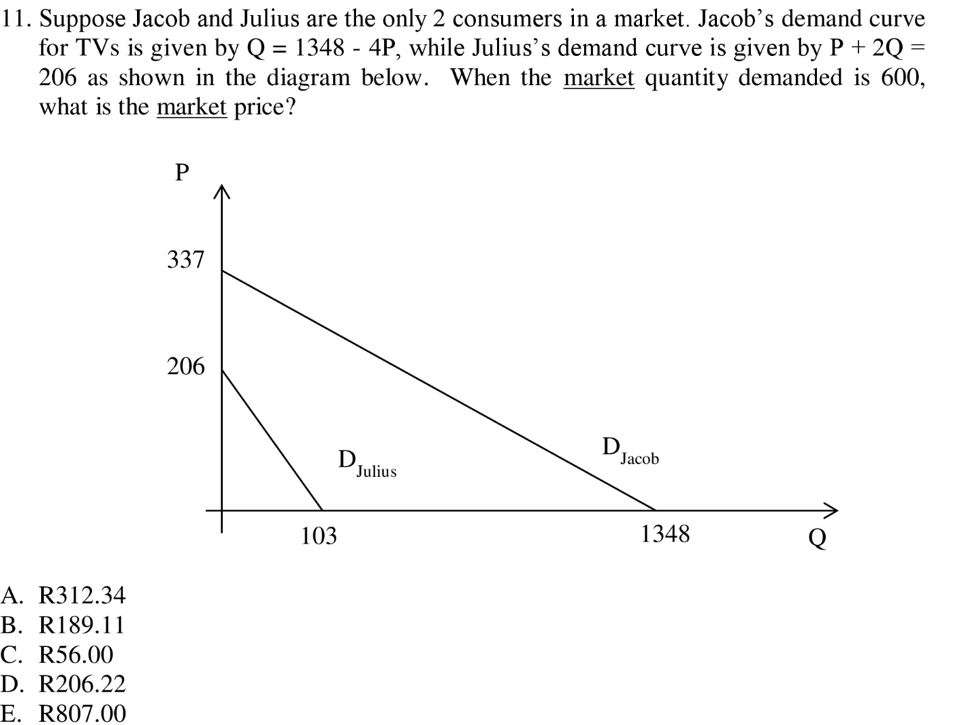# 11. Suppose Jacob and Julius are the only 2 consumers in a market. Jacob's demand curve for TVs is given by Q 1348 - 4P, while Julius's demand curve is given by P + 2Q 206 as shown in the diagram below. When the market quantity demanded is 600, what is the market price? 337 206 Jacob Julius 103 1348 A. R312.34 B. R189.11 C. R56.00 D. R206.22 E. R807.00

Questionhelp_outlineImage Transcriptionclose11. Suppose Jacob and Julius are the only 2 consumers in a market. Jacob's demand curve for TVs is given by Q 1348 - 4P, while Julius's demand curve is given by P + 2Q 206 as shown in the diagram below. When the market quantity demanded is 600, what is the market price? 337 206 Jacob Julius 103 1348 A. R312.34 B. R189.11 C. R56.00 D. R206.22 E. R807.00 fullscreen

### Want to see this answer and more?

Experts are waiting 24/7 to provide step-by-step solutions in as fast as 30 minutes!*